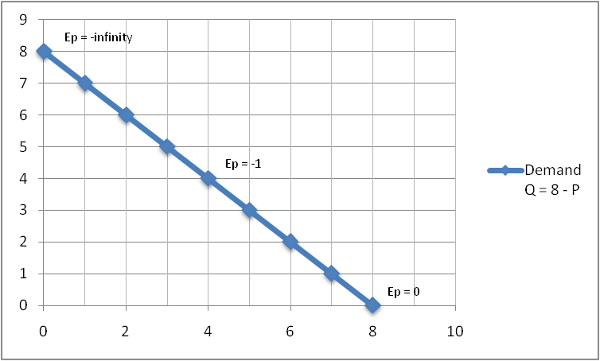## Price Elasticity

### Price Elasticity

Price elasticity measures the sensitivity of the quantity demanded or the quantity supplied to the change in the price. In other words, how much will a change in price affect the quantity demanded or supplied?

Price elasticity is calculated by taking the percentage change in quantity divided by the percentage change in price. On a linear supply or demand curve (a straight line), you can use the following price elasticity formulas:

Ep = (% Change Q) / (% Change P)
Ep = ((Change in Q) / (Q1)) / ((Change in P) / (P1))
Ep = ((Q2 - Q1) / (Q1)) / ((P2 - P1) / (P1))

Note that while price elasticity is related to the slope of the line, it is not actually the slope of the line. Recall that the slope of the line is calculated by "rise over run," or the change in the y-axis divided by the change in the x-axis. Price elasticity is calculated by "run over rise," or the change in quantity (on the x-axis) divided by the change in price (on the y-axis).

Generally, a curve is elastic if it is flat and more inelastic if it is more verticle. However, this can be a little misleading. Even on a linear (straight) demand or supply curve, the elasticity is not constant for the whole curve. The reason for this is that we are measuring the percentage change in both price and quantity. As you move along a linear curve and approach one of the axes, the percentage changes in that axis variable (either price or quantity) get smaller and smaller and the percentage changes of the opposite axis get bigger and bigger.

Price elasticity does NOT have a unit attached to it. That is, price elasticity is not measured in dollars or %, it is simply a ratio.

We should really look at price elasticity in two separate ways: the price elasticity of demand and the price elasticity of supply.

#### Price Elasticity of Demand

The first law of demand states that as price increases, less quantity is demanded. This is why the demand curve slopes down to the right. Because price and quantity move in opposite directions on the demand curve, the price elasticity of demand is always negative.

The image below shows the price elasticity of demand at different points along a simple linear demand curve, QD = 8 - P.Let's use the equation above, QD = 8 - P, to calculate the price elasticity of demand.

Imagine that the price is at 3, then moves up to 5. What is the elasticity?
At a price of 3, the quantity is 5 (Q = 8 - 3) and at a price of 5, the quantity is 3.
Ep = ((Q2 - Q1) / (Q1)) / ((P2 - P1) / (P1))
Ep = ((3 - 5) / (5)) / ((5 - 3) / 3)
Ep = -0.6

#### Price Elasticity of Supply

The typical supply curve is characterized by a line that slopes up to the right. Thus, as the price increases, more quantity is supplied. Because price and quantity move in the same directions on the supply curve, the price elasticity of supply is usually positive.

By B. Taylor, 2010

Home
Accounting
Economics
Economics Articles
Economic Data
Economics Glossary
Macroeconomics
Economic Indicators
Fiscal Policy
Microeconomics
The Supply Curve
Price Elasticity
Fixed & Variable Cost
ATC & Marginal Cost
Marginal Revenue
Output Decision
Price Floor
Price Ceiling
Negative Externalities
Positive Externalities
Price Gouging
Sunk Costs
Game Theory
Game Theory Introduction
Nash Equilibrium
Extensive Form
Monopolies
Monopolies
The Sherman Act
The Clayton Act
Papers
Bootstrap Method
The CAPM Model
Euro Creation and Crisis
FX and Inflation in Pakistan
Poverty and Crime
General Finance
Mergers / Acquisitions
Money Market
Options
Real Estate
Retirement
Stocks
Other Personal Finance
Opinions / Essays
Excel